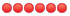NEVER post copyrighted questions. Always state where questions come from. We take copyright violations very serious. Any copyrighted materials will be removed and the posters account will be deactivated.
 Page:1

# TOPIC: Free PMP® Exam Sample Question of the Week

## Free PMP® Exam Sample Question of the Week 9 months 5 days ago #17507

 Mary Kathrine PaduaTopic Author Offline AdministratorPosts: 211 Karma: 7 Thank you received: 99Hello everyone! Here is this week's free PMP® exam sample question from the PM Exam Simulator. Your sponsor has committed to building a high-speed rail from one major inland city, Azules, to the coast. You have two possible routes, either Brazos or Corazon. Given each route's costs, odds, and rewards of high demand and low demand in this decision tree, how would you quantify the expected monetary value of the route to Brazos? A. 1.6 billion B. -2 billion C. 0.8 billion D. 10.8 billion HINT: The expected monetary value is an important calculation in performing decision tree analysis as part of the Perform Quantitative Risk Analysis process. You must account for not only the cost of the decision, but the probability and payoff for each effect. All our questions are updated to the latest A Guide to the Project Management Body of Knowledge (PMBOK® Guide) standard. Stop by at free.pm-exam-simulator.com/ and try the PM Exam Simulator free for 7 days. We are a Project Management Institute (PMI)® Registered Education Provider. Answer and Explanation: The correct answer is C. The expected monetary value is an important calculation in performing decision tree analysis as part of the Perform Quantitative Risk Analysis process. You must account for not only the cost of the decision but the probability and payoff for each path. In a decision tree, the EMV for a decision is calculated by multiplying the value of each possible profit outcome by its probability of occurrence and adding the products together. The value of each possible outcome is the net path value. Remember: when you net something, you should consider both positive and negative flows. The formula to compute net path value is to subtract the path reward minus the cost of its decision. For building the route to Brazos, here are the steps to compute the expected monetary value: EMV = 0.8(12B - 10B)] + 0.2(6B - 10B) EMV = 0.8(2B) + 0.2(-4B) EMV = 1.6B - 0.8B EMV = 0.8B Other answer choices do not properly factor the net path value (the payoff minus the cost of the decision) and sum the effects. Below is the completed decision tree with all the net path values and the resulting expected monetary value of each choice. The EMV for the Brazos route is 0.8 billion, and the EMV for the Corazon route is -5.2 billion (note this is a negative number). Since the path with the largest expected monetary value is the Brazos route at .8 billion, that is the best choice. Details for each option: A. 1.6 billion Incorrect. You might choose this answer if you merely multiply one path's chance by its net path value. For each decision branch, all effects are added to determine the expected monetary value. Even if you chose the most valuable path, the expected monetary value in a decision tree must include all effects. B. -2 billion Incorrect. You might choose this answer if you merely added the net path values. However, to compute the expected monetary value, you must factor the probability of each payoff occurring. C. 0.8 billion Correct. To calculate the expected monetary value of the route to the Brazos, you multiply each path's chance by its net path value and sum the results. EMV = 0.8(2B) + 0.2(-4B) = 1.6B + -0.8B = 0.8B D. 10.8 billion Incorrect. You might have chosen this answer if you merely multiplied the probability by the payoff, e.g. (0.8*12+0.2*6). However, you must also account for the cost of the decision to build the route to Brazos, so this is not the best answer. Reference: A Guide to the Project Management Body of Knowledge (PMBOK® Guide) – Sixth Edition, Project Management Institute Inc., 2017, Page(s) 435 Your e-mail address will never be displayed on the site. Check this box to be notified of replies to this topic. Note: BBcode and smileys are still usable. Last edit: by Mary Kathrine Padua.
 Page:1
Moderators: Yolanda MabutasScott GillardMary Kathrine PaduaTracy Shagnea, PMPJoe PangJohn Paul BugarinAmy MartinezLisa Sweeney PMP

OSP INTERNATIONAL LLC
OSP INTERNATIONAL LLC
Training for Project Management Professional (PMP)®, PMI Agile Certified Practitioner (PMI-ACP)®, and Certified Associate in Project Management (CAPM)®